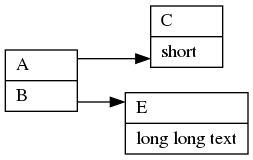# Is there a way to justify/align nodes on the same rank?

Dot aligns (justifies) all the nodes on the same rank to a common Y value (or common X value if rankdir=LR/RL)
For example the two nodes on the right side are on the same rank and share a common X value:Is there a (built-in) way to left-justify the two nodes?

This is a great idea, but we did not implement this. I wonder how hard it would be - there might be some code that assumes node center points are on the midline of a rank, but I don’t think that’s baked in very deeply. Think the spline router already depends on a function that determines for each node, the box path from an edge port to the inter-rank space where the spline is going next, so this feature would fit in with that already.

Here is a gvpr (https://www.graphviz.org/pdf/gvpr.1.pdf) program that will (re)justify/align nodes within their given rank.

use it like this:

• create a dot file as normal
• add this new attribute to all nodes you want justified rankjustify= X where X can be t|b|l|r|c|max|min. Different nodes can have different rankjustify values.
• run dot, gvpr, and neato to produce the desired graph
• `dot -Tdot myfile.gv | gvpr -cf rankJustify.gvpr | neato -n -Tpng >myfile.png`

Here is rankJustify.gvpr:

``````//
// justify nodes that are on the same rank
//
BEGIN {
int nxt=0, i, vert, rankPos[], OK[], checked[];
string RankDir;
float num, deltaX, deltaY, maxHeight[], maxWidth[];
graph_t aGraph, Root, Parent[];
node_t  aNode;
///////////////////////////////////////////////////////////////////////////////
void nodeJustify(node_t aN){
float XX, YY;
int err;

if(checked[aN]==1) return;
checked[aN]=1;
if (! hasAttr(aN, "rankjustify") || aN.rankjustify=="") continue;
XX=aN.X;
YY=aN.Y;
deltaX=0.;
deltaY=0.;
if (vert==1){
deltaY=72.*(maxHeight[aN.Y]-(float)aN.height)/2.;  // inches to points
}else{
deltaX=72.*(maxWidth[aN.X]-(float)aN.width)/2.;    // inches to points
}
aN.oldPos=aN.pos;
switch(OK[aN.rankjustify]){
case "1":
break;
case "-1":
deltaX=-deltaX;
deltaY=-deltaY;
break;
case "0":
deltaX=0.;
deltaY=0.;
break;
default:
print("//  Error:: node ",aN.name, " has invalid rankjustify value (", aN.rankjustify,")");
printf(2, "Error:: node %s, has invalid rankjustify value (%s)\n", aN.name, aN.rankjustify);
continue 2;  // exit two levels
break;
}
aN.pos=sprintf("%.2f,%.2f", (aN.X + deltaX), (aN.Y + deltaY));
if (hasAttr(aN,"xlp") && aN.xlp!="") {
sscanf (aN.xlp, "%lf,%lf", &XX, &YY);
aN.xlp=sprintf("%.2f,%.2f", (XX + deltaX), (YY + deltaY));
}
}
///////////////////////////////////////////////////////////////////////////////
void checkaGraph(graph_t aGraph){
for (aNode=fstnode(aGraph);aNode;aNode = nxtnode_sg(aGraph, aNode)){
Parent[aNode]=aGraph;
// first pass through the nodes
// find max width/height for each rank/cluster (based on common Y or X)
if (RankDir=="TB|BT"){
rankPos[aNode.Y]=1;
if (maxHeight[aNode.Y]<aNode.height){
maxHeight[aNode.Y]=aNode.height;
}
} else {   // LR or RL
rankPos[aNode.X]=1;
if (maxWidth[aNode.X]<aNode.width)
maxWidth[aNode.X]=aNode.width;
}
}
for (aNode=fstnode(aGraph);aNode;aNode = nxtnode_sg(aGraph, aNode)){
nodeJustify(aNode);
}
}
///////////////////////////////////////////////////////////////////////////////
graph_t graphTraverse(graph_t thisG){
for (aGraph = fstsubg(thisG); aGraph; aGraph = nxtsubg(aGraph)) {
if (match(aGraph.name,"cluster")==0 || (hasAttr(aGraph, "cluster") && aGraph.cluster=="true")){
unset(maxWidth);
unset(maxHeight);
checkaGraph(aGraph);
}
aGraph = graphTraverse(aGraph);
}
return thisG;
}  // end of graphTraverse
}
BEG_G{
Root=\$G;
if (hasAttr(Root, "layout")){
// other values (including "") cause problems with later execution
Root.layout="neato";
}
if (! hasAttr(Root, "splines") || Root.splines==""){
// dot defaults to true, neato defaults to false
Root.splines="true";
}
//  determine acceptable justification values
if (! hasAttr(\$G, "rankdir") || \$.rankdir==""){
RankDir="TB";
}else{
RankDir=\$G.rankdir;
}
vert=1;
OK["c"]   =0;
if (RankDir=="TB") {
OK["t"]   =1;
OK["b"]   =-1;
//OK["max"] =1;
//OK["min"] =-1;
OK["max"] =-1;
OK["min"] =1;
OK["l"]   =1;
OK["r"]   =-1;
}  else if (RankDir=="BT"){
OK["t"]   =1;
OK["b"]   =-1;
//OK["max"] =-1;
//OK["min"] =1;
OK["max"] =1;
OK["min"] =-1;
// do we really want these next two?
OK["l"]   =1;
OK["r"]   =-1;
}  else if (RankDir=="LR"){
vert=0;
OK["l"]   =-1;
OK["r"]   =1;
//OK["max"] =-1;
//OK["min"] =1;
OK["max"] =1;
OK["min"] =-1;
// do we really want these next two?
OK["t"]   =-1;
OK["b"]   =1;
}  else if (RankDir=="RL"){
vert=0;
OK["l"]   =-1;
OK["r"]   =1;
//OK["max"] =1;
//OK["min"] =-1;
OK["max"] =-1;
OK["min"] =1;
}
// traverse the graph
//   find all clusters
//      within each cluster, find all nodes (at highest level
//        find max & min Y (or X) within the set of nodes
//        then revisit all of the nodes and adjust Y (or X) as desired
// (finally) for all nodes not visited
//        find max & min Y (or X) within the set of nodes
//        then revisit all of the nodes and adjust Y (or X) as desired
graphTraverse (Root);
unset(maxWidth);
unset(maxHeight);
checkaGraph(Root);
}
``````

And an example of the result:

2 Likes